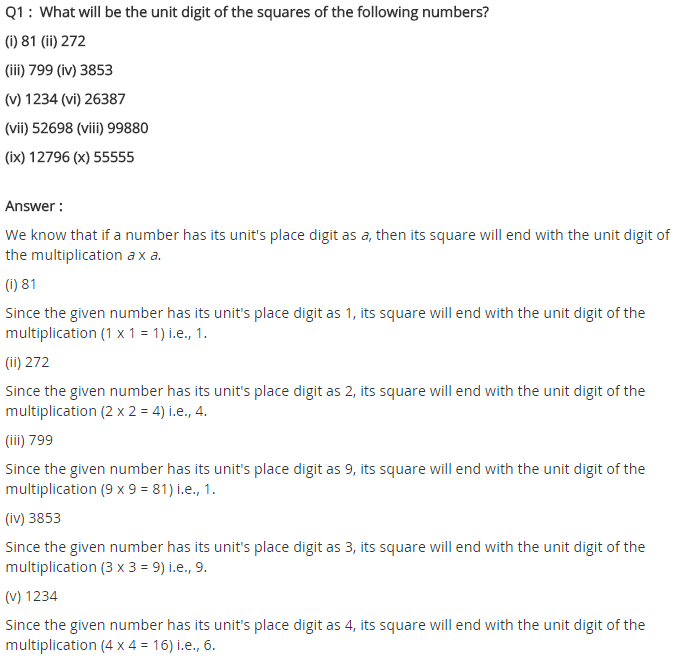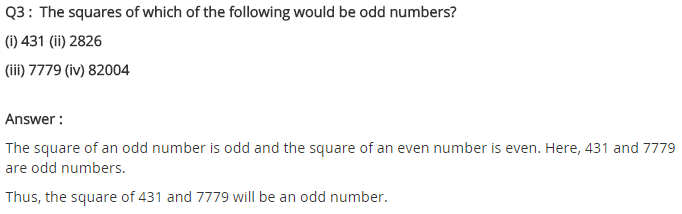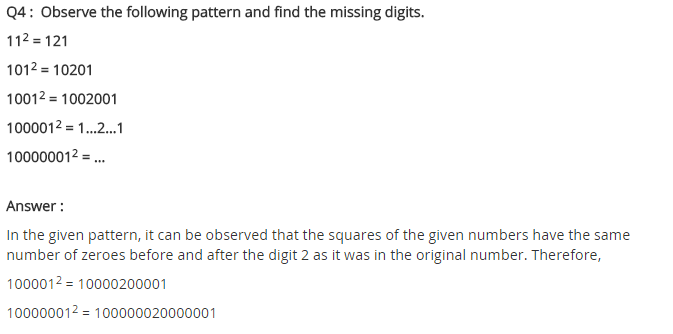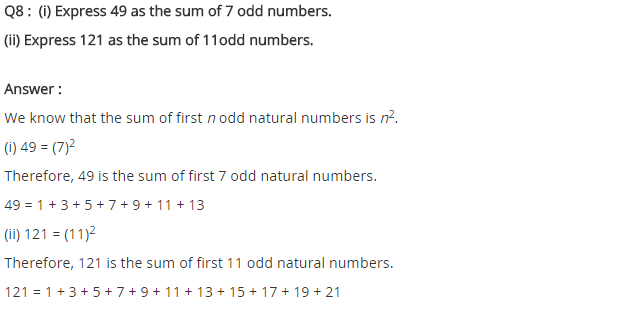# NCERT Solutions for Class 8 Maths Chapter 6 Squares and Square Roots

NCERT Solutions for Class 8 Maths Chapter 6 Squares and Square Roots

### NCERT Solutions for Class 8 Maths Chapter 6 Squares and Square Roots Exercise 6.1

Ex 6.1 Class 8 Maths Question 1.
What will be the unit digit of the squares of the following numbers?
(i) 81
(ii) 272
(iii) 799
(iv) 3853
(v) 1234
(vi) 20387
(vii) 52698
(viii) 99880
(ix) 12796
(x) 55555
Solution:
(i) Unit digit of 812 = 1
(ii) Unit digit of 2722 = 4
(iii) Unit digit of 7992 = 1
(iv) Unit digit of 38532 = 9
(v) Unit digit of 12342 = 6
(vi) Unit digit of 263872 = 9
(vii) Unit digit of 526982 = 4
(viii) Unit digit of 998802 = 0
(ix) Unit digit of 127962 = 6
(x) Unit digit of 555552 = 5

Ex 6.1 Class 8 Maths Question 2.
The following numbers are not perfect squares. Give reason.
(i) 1057
(ii) 23453
(iii) 7928
(iv) 222222
(v) 64000
(vi) 89722
(vii) 222000
(viii) 505050
Solution:
(i) 1057 ends with 7 at unit place. So it is not a perfect square number.
(ii) 23453 ends with 3 at unit place. So it is not a perfect square number.
(iii) 7928 ends with 8 at unit place. So it is not a perfect square number.
(iv) 222222 ends with 2 at unit place. So it is not a perfect square number.
(v) 64000 ends with 3 zeros. So it cannot a perfect square number.
(vi) 89722 ends with 2 at unit place. So it is not a perfect square number.
(vii) 22000 ends with 3 zeros. So it can not be a perfect square number.
(viii) 505050 ends with 1 zero. So it is not a perfect square number.

Ex 6.1 Class 8 Maths Question 3.
The squares of which of the following would be odd numbers?
(i) 431
(ii) 2826
(iii) 7779
(iv) 82004
Solution:
(i) 4312 is an odd number.
(ii) 28262 is an even number.
(iii) 77792 is an odd number.
(iv) 820042 is an even number.

Ex 6.1 Class 8 Maths Question 4.
Observe the following pattern and find the missing digits.
112 = 121
1012 = 10201
10012 = 1002001
1000012 = 1…2…1
100000012 = ………
Solution:
According to the above pattern, we have
1000012 = 10000200001
100000012 = 100000020000001

Ex 6.1 Class 8 Maths Question 5.
Observe the following pattern and supply the missing numbers.
112 = 121
1012 = 10201
101012 = 102030201
10101012 = ……….
……….2 = 10203040504030201
Solution:
According to the above pattern, we have
10101012 = 1020304030201
1010101012 = 10203040504030201

Ex 6.1 Class 8 Maths Question 6.
Using the given pattern, find the missing numbers.
12 + 22 + 22 = 32
22 + 32 + 62 = 72
32 + 42 + 122 = 132
42 + 52 + ….2 = 212
52 + ….2 + 302 = 312
62 + 72 + …..2 = ……2
Solution:
According to the given pattern, we have
42 + 52 + 202 = 212
52 + 62 + 302 = 312
62 + 72 + 422 = 432

Ex 6.1 Class 8 Maths Question 7.
(i) 1 + 3 + 5 + 7 + 9
(ii) 1 + 3 + 5 + 7 + 9 + 11 + 13 + 15 + 17 + 19
(iii) 1 + 3 + 5 + 7 + 9 + 11 + 13 + 15 + 17 + 19 + 21 + 23
Solution:
We know that the sum of n odd numbers = n2
(i) 1 + 3 + 5 + 7 + 9 = (5)2 = 25 [∵ n = 5]
(ii) 1 + 3 + 5 + 7 + 9 + 11 + 13 + 15 + 17 + 19 = (10)2 = 100 [∵ n = 10]
(iii) 1 + 3 + 5 + 7 + 9 + 11 + 13 + 15 + 17 + 19 + 21 + 23 = (12)2 = 144 [∵ n = 12]

Ex 6.1 Class 8 Maths Question 8.
(i) Express 49 as the sum of 7 odd numbers.
(ii) Express 121 as the sum of 11 odd numbers.
Solution:
(i) 49 = 1 + 3 + 5 + 7 + 9 + 11 + 13 (n = 7)
(ii) 121 = 1 + 3 + 5 + 7 + 9 + 11 + 13 + 15 + 17 + 19 + 21 (n = 11)

Ex 6.1 Class 8 Maths Question 9.
How many numbers lie between squares of the following numbers?
(i) 12 and 13
(ii) 25 and 26
(iii) 99 and 100.
Solution:
(i) We know that numbers between n2 and (n + 1)2 = 2n
Numbers between 122 and 132 = (2n) = 2 × 12 = 24
(ii) Numbers between 252 and 262 = 2 × 25 = 50 (∵ n = 25)
(iii) Numbers between 992 and 1002 = 2 × 99 = 198 (∵ n = 99)## SabDekho

The Complete Educational Website